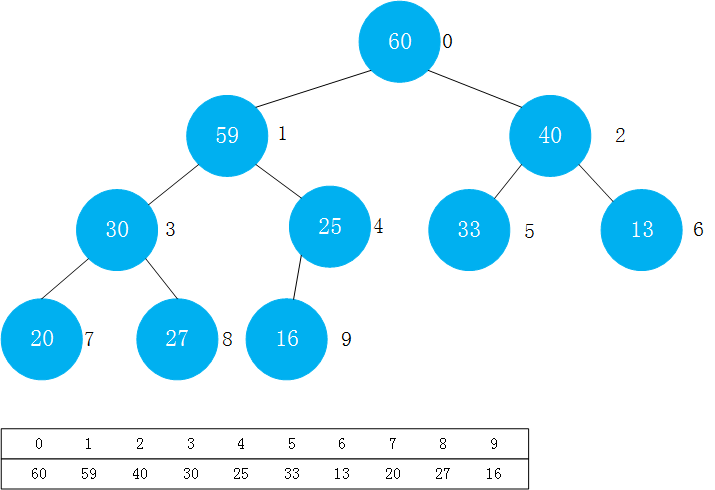O(logn) O(logn)

# 堆

• 堆中某个节点的值总是不大于或不小于其父节点的值(所以分为最大堆和最小堆)
• 堆总是一棵完全二叉树

## 堆的表示方法——动态数组``````public class MaxHeap<E extends Comparable<E>> {
private Array<E> data;

public MaxHeap(int capacity){
data = new Array<>(capacity);
}

public MaxHeap(){
data = new Array<>();
}

//返回堆中的元素个数
public int size() {
return data.getSize();
}

//判断堆是否为空
public boolean isEmpty() {
return data.isEmpty();
}

//返回一个完全二叉树的数组表示中，一个索引所表示的元素的父亲节点的索引
private int parent(int index){
if(index == 0)
throw new IllegalArgumentException("index 0 doesn't have parent;");
return (index-1) / 2;
}

//返回一个完全二叉树的数组表示中，一个索引所表示的元素的左孩子的索引
private int leftChild(int index){
return index * 2 + 1;
}

//返回一个完全二叉树的数组表示中，一个索引所表示的元素的右孩子的索引
private int rightChild(int index){
return index * 2 + 2;
}

//向堆中添加一个元素
//调用siftUp函数，进行上浮操作,以维护堆的性质
siftUp(data.getSize() - 1);
}

//需要上浮的元素的索引
private void siftUp(int k){
//如果k满足索引大于零,且其父亲节点的值小于它的值，则进行上浮操作
while (k > 0 && data.get(parent(k)).compareTo(data.get(k)) < 0){
swap(k,parent(k));
k = parent(k);
}
}

//定义一个交换的函数
private void swap(int i , int j){
if(i < 0 || i >= data.getSize() || j < 0 || j>= data.getSize()){
throw new IllegalArgumentException("Index is illegal.");
}
E temp = data.get(i);
data.set(i,data.get(j));
data.set(j,temp);
}

//查看堆中的最大元素
public E findMax(){
if(data.getSize() == 0)
throw new IllegalArgumentException("Can not findMac in a empty heap");
return data.get(0);
}

//取出堆中的最大元素
public E extractMax(){
E ret = findMax();

swap(0,data.getSize() - 1);
data.removeLast();
siftDown(0);

return ret;
}

//下沉操作
private void siftDown(int k){
while (leftChild(k) < data.getSize()){//如果k没有左右孩子，则循环终止

int j = leftChild(k);//j为k的左孩子的索引
if(j + 1 < data.getSize() && data.get( j + 1 ).compareTo(data.get(j)) > 0){//如果k有右孩子,并且右孩子的节点的值大于左孩子的节点的值
j = rightChild(k);
//此时，data[j]是leftChild和rightChild中的最大值
}
if(data.get(k).compareTo(data.get(j)) >= 0) // 如果k的值大于其左右孩子的值，则满足了最大堆的另一个性质，可以退出循环体
break;
//否则交换k和j位置,并且k赋值为j，继续进行下一个循环
swap(k,j);
k = j;
}
}
}
``````
• 在数组中，一个父亲节点其所有节点的索引(假设索引以0开始)为：
``````Index_leftChild  = index_Father * 2 + 1;
Index_rightChild = index_Father * 2 + 2;
``````
• 如果知道一个孩子节点的索引(假设索引以0开始)求其父亲节点的索引:
``````index_Father = ( index_Child - 1 ) / 2;
``````

上面两个求法可以用数学归纳法进行证明。

• `Sift Up`操作很简单，即新添加的元素先放到数组的末尾位置，这时候满足了完全二叉树的性质。但是它不一定满足总是不大于或者不小于其父亲节点的值。所以这时候的操作是，这个值要与它的父亲节点、爷爷节点……对比，直到放在合适的位置。因为方法中总结了找到一个节点的父亲节点的方法:`faterIndex = (index-1) / 2`。以一个最大堆为例，让新加入的节点和父亲节点对比，如果它大于其父亲节点，则交换，继续对比其父亲节点，直到它小于等于其父亲节点为止。

• `Sift Down`操作，即取出最大堆的堆顶的元素（取出操作只能取出这个最大的元素，而不能取出别的元素）。因为最大的元素取出后，其左右的树结构就是两个单独的子树，那么要给这两个子树找一个新的父节点，操作如下：
1. 将堆中的最后一个元素放在堆顶
2. 删除掉最后一个元素（这时候满足完全二叉树的性质）
3. 将堆顶元素与左右孩子中`大于它``较大`的数进行交换
4. 交换后的新位置继续与孩子节点中`大于它``较大`的数进行交换
5. 继续操作直到它大于其左后孩子或者它没有左右孩子

• 其中`swap`交换函数我写在了堆这个类中，可以在Array类中定义交换函数，在堆类中直接调用即可。

## Heapify 和 replace操作

### replace

replace：取出最大元素后放入新元素

• step1: extractMax -> step2: add (2O(logn))
• setp1: 替换堆顶元素 -> step2: Sift Down (O(logn))
``````//取出堆中的最大元素，并替换成e
public E replace(E e){
E ret = findMax();
data.set(0,e);
siftDown(0);
return ret;
}
``````

### Heapify

将任意数组整理成堆的形状

• 扫描数组，放如堆的新的对象中再返回 (O(nlogn))
• 可以先把数组看成一棵完全二叉树,从最后一个非叶子节点开始进行`Sift Down`操作。(找到最后一个非叶子节点的方法：拿到最后一个节点的索引，然后计算他的父亲节点的索引即可) step1: 找到最后一个非叶子节点，进行`Sift Down`操作 step2: 倒数第二个非叶子节点进行`Sift Down`操作 … 以此类推 直到索引为0的非叶子节点完成`Sift Down`操作 O(n)
• Heapify一般可以在构造函数中进行(用一个数组初始化堆)),所以Array类也要支持一个用数组初始化动态数组的构造函数

Array类的构造函数：

``````public Array(E[] arr)  {
data = (E[])new Object[arr.length];
for(int i = 0 ; i < arr.length ; i ++){
data[i] = arr[i];
}
size = arr.length;
}

``````

MaxHeap的构造函数：

``````public MaxHeap(E[] arr){
data = new Array<>(arr);
for(int i = parent(arr.length - 1) ; i >= 0 ; i--)
siftDown(i);
}
``````

# 优先队列

``````public class PriorityQueue<E extends Comparable<E>> implements Queue<E> {
private MaxHeap<E> maxHeap;
public PriorityQueue(){
maxHeap = new MaxHeap<>();
}
@Override
public int getSize() {
return maxHeap.size();
}

@Override
public boolean isEmpty() {
return maxHeap.isEmpty();
}

@Override
public void enqueue(E e) {
}

@Override
public E dequeue() {
return maxHeap.extractMax();
}

@Override
public E getFront() {
return maxHeap.findMax();
}
}
``````
• java.util中的PriorityQueue默认是用的最小堆，具体方法名也有一些区别